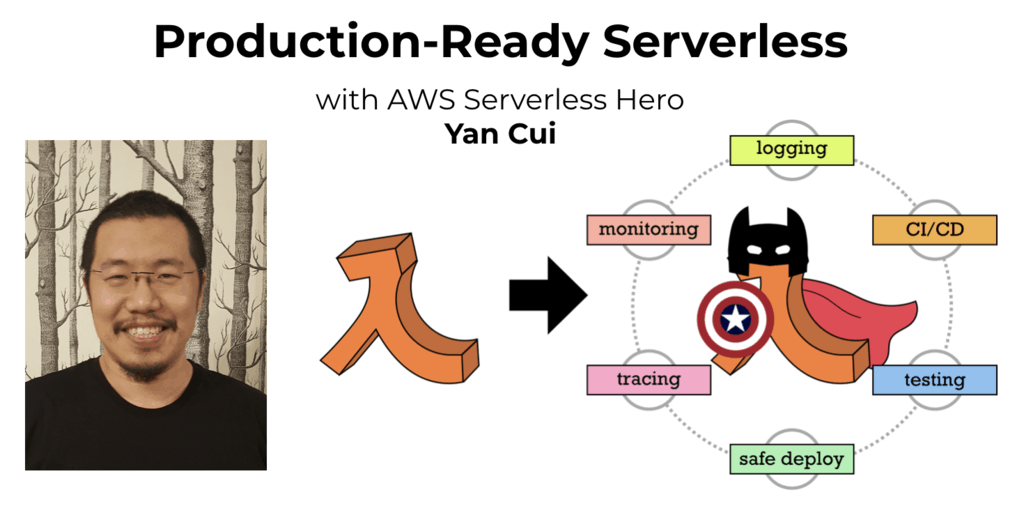# Project Euler – Problem 46 Solution

Check out my new course Learn you some Lambda best practice for great good! and learn the best practices for performance, cost, security, resilience, observability and scalability.

#### Problem

It was proposed by Christian Goldbach that every odd composite number can be written as the sum of a prime and twice a square.

9 = 7 + 2×12

15 = 7 + 2×22

21 = 3 + 2×32

25 = 7 + 2×32

27 = 19 + 2×22

33 = 31 + 2×12

It turns out that the conjecture was false.

What is the smallest odd composite that cannot be written as the sum of a prime and twice a square?

#### Solution

```let hasDivisor(n) =
let upperBound = int64(sqrt(double(n)))
[2L..upperBound] |> Seq.exists (fun x -> n % x = 0L)

// need to consider negative values
let isPrime(n) = if n <= 1L then false else not(hasDivisor(n))

// generate the sequence of odd composite numbers
let oddCompositeNumbers =
Seq.unfold (fun state -> Some(state, state+2L)) 9L
|> Seq.filter (fun n -> not(isPrime n))

// generate the sequence of prime numbers
let primeNumbers = Seq.unfold (fun state -> Some(state, state+2L)) 1L |> Seq.filter isPrime

// function to check if a number can be written as the sum of a prime and twice a square
let isSum(number) =
|> Seq.takeWhile (fun n -> n < number)
|> Seq.exists (fun n -> sqrt(double((number-n)/2L)) % 1.0 = 0.0)

let answer = oddCompositeNumbers |> Seq.filter (fun n -> not(isSum(n))) |> Seq.head
```

All pretty straight forward here, the only slightly confusing part of this solution is how to determine if a number can be written as the sum of a prime and twice a square:

Odd Composite Number = Prime + 2 x n2 => n = sqrt((Odd Composite Number – Prime) / 2)

As you know Math.Sqrt works with a double and returns a double, hence to find out if n above is a whole number I had to check whether it divides by 1 evenlyHi, I’m Yan. I’m an AWS Serverless Hero and the author of Production-Ready Serverless.

I specialise in rapidly transitioning teams to serverless and building production-ready services on AWS.

Are you struggling with serverless or need guidance on best practices? Do you want someone to review your architecture and help you avoid costly mistakes down the line? Whatever the case, I’m here to help.Check out my new course, Learn you some Lambda best practice for great good! In this course, you will learn best practices for working with AWS Lambda in terms of performance, cost, security, scalability, resilience and observability. Enrol now and enjoy a special preorder price of £9.99 (~\$13).Are you working with Serverless and looking for expert training to level-up your skills? Or are you looking for a solid foundation to start from? Look no further, register for my Production-Ready Serverless workshop to learn how to build production-grade Serverless applications!